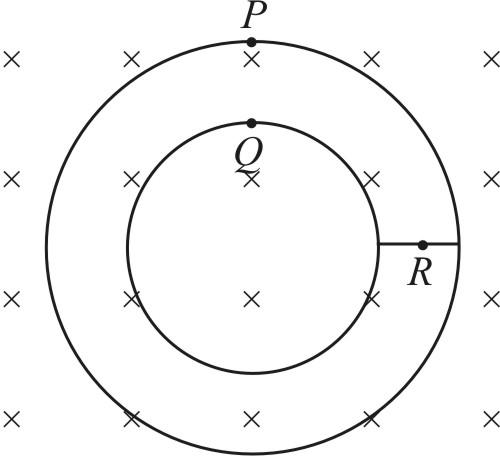Question of Exercise 1

# Question Figure shown plane figure made of a conductor located in a magnetic field along the inward normal to the plane of the figure. The magnetic field starts diminishing. Then the induced currentOption 1 at point P is clockwise

Option 2 at point Q is anticlockwise

Option 3 at point Q is clockwise

Option 4 at point R is zero

State and explain Coulombs inverse square law in electricity

Solution:

Explanation:

According to Coulomb's inverse square law, the electrostatic force generated due to two charges placed at a distance is

inversely proportional to the square of the distance between these two charges.If we apply a force in a straight line to the charges that are repulsive, the force will be repulsive, and if the charges are opposite, the force will be attractive.

Hence, according to Coulomb's inverse square law in electricity.

The substances which are attracted towards a magnet are called

A: metals

B: ferromagnetic materials

C: non - metals

D: diamagnetic materials

Solution:

Explanation:

Metals are good conductors of heat and electricity. Iron gets attracted by the magnet and it is metal but all metals are not attracted by the magnets.

Ferromagnetic materials are the materials that get attracted by the magnet.

Non-metals do not have any magnetizing properties.

Materials that do not get attracted by magnets and cannot be magnetized as well are known as diamagnetic materials.

Hence, option B is correct.

A magnetic field exerts no force on

A: a stream of electrons

B: a stream on protons

C: unmagnetised piece of iron

D: stationary charge.

Solution:

Explanation:

A magnetic field exerts no force on stationary charge because a stationary charge does not have the ability to develop magnetic field or get affected by any external magnetic field. A moving charge is responsible for the generation of current in the circuit which in result generates the magnetic field.

Hence, option D is correct.

Name the material which is the best conductor of electricity

Solution:

Explanation:

The best conductor of electricity is silver metal because of its crystal structure. Thus, silver has the highest number of free electrons than other metals but as it is very expensive in comparison to copper and aluminum, it is not used for making wires.

Hence, the material which is the best conductor of electricity is silver.

Which quantity is increased in the step-down transformer

A: Current

B: Voltage

C: Power

D: Frequency

Solution:

Explanation:

In a step-down transformer, the voltage is decreased and the current is increased.